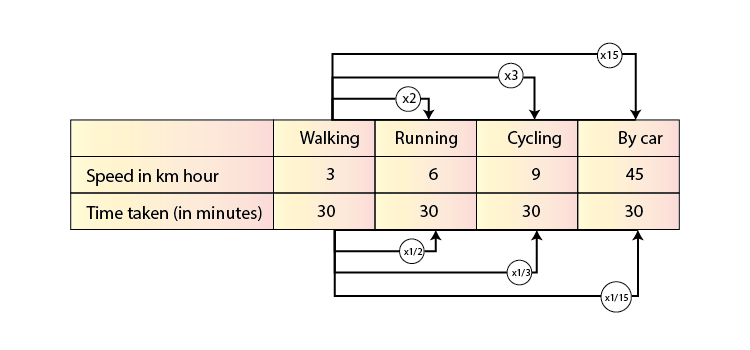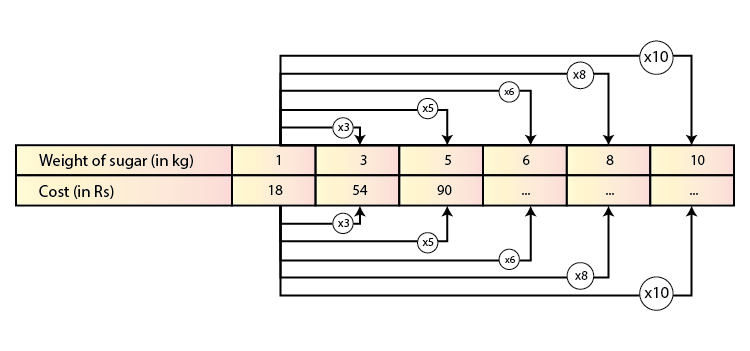# Direct and Inverse Proportions Class 8 Maths Notes Chapter 13

According to the CBSE Syllabus 2023-24, this chapter has been renumbered as Chapter 11.

When two variables change in the same sense i.e., as one amount increases, the other amount also increases at the same rate it is called direct proportionality. When two variables such as x and y are given, y is directly proportional to x if there is a non-zero constant k. The constant ratio is called the constant of proportionality or proportionality constant.

## Inverse Proportions

If the value of variable x decreases or increases upon corresponding increase or decrease in the value of variable y, then we can say that variables x and
y are in inverse proportion.

For example : In the table below, we have variable y: Time taken (in minutes) reducing proportionally to the increase in value of variable x: Speed (in km/hour). Hence the two variables are in inverse proportion.To know more about Direct and Inverse Proportion, visit here.

### Relation for Inverse Proportion

Considering two variables x and y,
xy=k or x=

$$\begin{array}{l}\frac{k}{y}\end{array}$$
establishes the relation for inverse proportionality between x and y, where k is a constant.

So if x and y are in inverse proportion, it can be said that

$$\begin{array}{l}\frac{x_{1}}{x_{2}}\end{array}$$
=
$$\begin{array}{l}\frac{y_{2}}{y_{1}}\end{array}$$
where y1 and y2 are corresponding values of variables x1 and x2

### Time and Work

It is important to establish the relationship between time taken and the work done in any given problem or situation. If time increases with increase in work, then the relation is directly proportional. In such a case we will use

$$\begin{array}{l}\frac{x_{1}}{y_{1}}\end{array}$$
=
$$\begin{array}{l}\frac{x_{2}}{y_{2}}\end{array}$$

to arrive at our solution.

However if they are inversely proportional we will use the relation

$$\begin{array}{l}\frac{x_{1}}{x_{2}}\end{array}$$
=
$$\begin{array}{l}\frac{y_{2}}{y_{1}}\end{array}$$

For example: In the table below, we have the number of students (x) that took a  certain number of days (y) to complete a fixed amount of food supplies. Now we have to calculate the number of days it would take for an increased number of students to finish the identical amount of food.

 Number of students 100 125 Number of days 20 y

We know that with greater number of people, the time taken to complete the food will be lesser, therefore we have an inverse proportionality relation between x and y here.

Hence by applying the formula, we have:

$$\begin{array}{l}\frac{100}{125}\end{array}$$
=
$$\begin{array}{l}\frac{y}{20}\end{array}$$
⇒ =
$$\begin{array}{l}\frac{20.100}{125}\end{array}$$

=16 days

## Introduction to Direct Proportions

### Direct Proportion

If the value of a variable x always increases or decreases with the respective increase or decrease in value of variable y, then it is said that the variables x and y are in direct proportion.

For example : In the table below, we have variable y – Cost (in Rs) always increasing when there is an increase in variable x – Weight of sugar (in kg). Likewise if the weight of sugar reduced, the cost would also reduce. Hence the two variables are in direct proportion.To know more about Direct Proportion, visit here.

### Relation for Direct Proportion

Considering two variables x and y,

$$\begin{array}{l}\frac{x}{y}\end{array}$$
k or x=ky establishes the simple relation for direct proportion between x and y, where k is a constant.

So if x and y are in direct proportion, it can be said that

$$\begin{array}{l}\frac{x_{1}}{y_{1}}\end{array}$$
=
$$\begin{array}{l}\frac{x_{2}}{y_{2}}\end{array}$$
where y1 and y2 correspond to respective values of x1 and x2.

## Frequently asked Questions on CBSE Class 8 Maths Notes Chapter 13 Direct and Inverse Proportions

Q1

### What is a Direct Proportion?

Direct proportion or direct variation is the relation between two quantities where the ratio of the two is equal to a constant value.

Q2

### What is an Inverse Proportion?

Inverse proportion occurs when one value increases and the other decreases.

Q3

### What are the uses of Time and Work problems?

Time and work problems are important because there is a certain relationship between the number of persons doing the work, number of days or time taken by them to complete the work and the amount of work that is done.